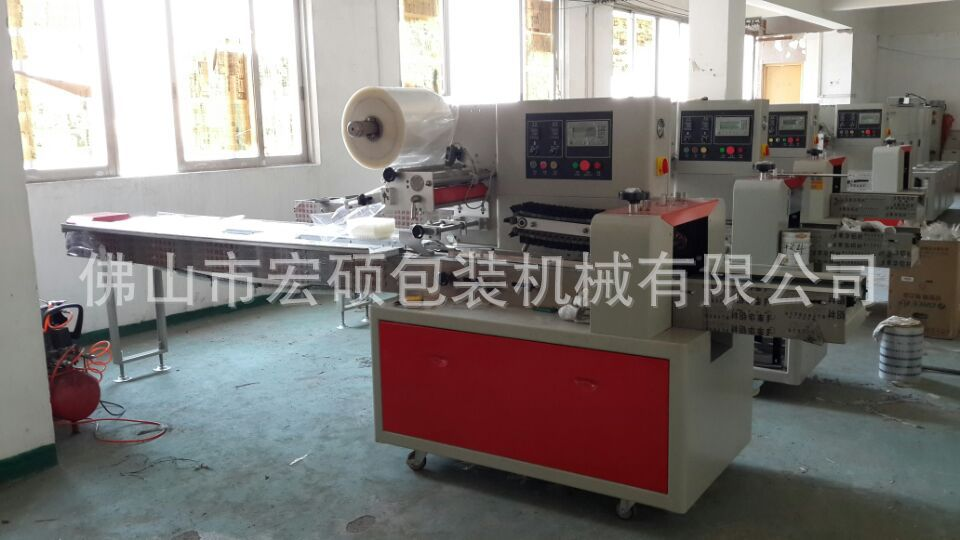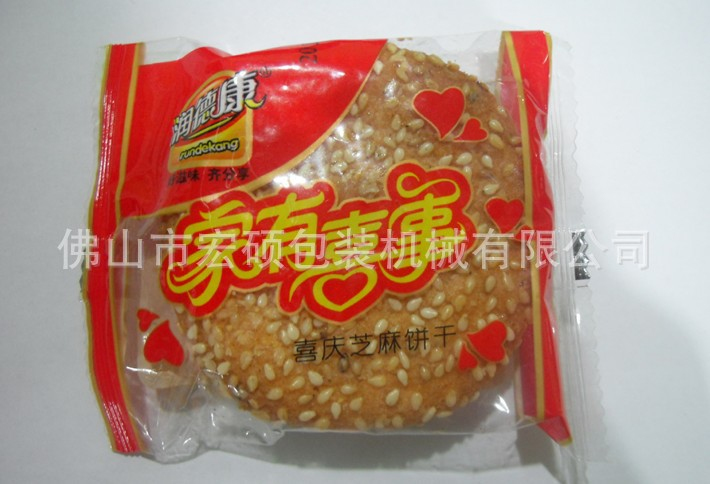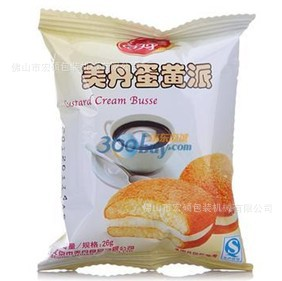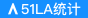# 自动包装机械,专业包装机械,专业机械,定制包装机械,定制机械,

• 价格要求： 电议
• 采购数量：1000
• 包装要求：无要求
• 采购情况：长期有效
• 所在地：广东
• 发布日期：2020-08-20 23:56
• 信息有效期： 发布者未注册，不计时。登录后方可查看联系方式

• 所在地：广东
• 有效期至：长期有效
• 浏览次数：759

r r r r r r r
r r r r r r r r
 r r 售后服务：凡购买本公司的产品后,我们派专业技术人员到贵公司安装，调试及操作培训，至贵公司技术工能熟练操作。我公司的所有产品从你购买日起，保修期为一年，在保修期内，我公司派专业技术人员及时上门无偿维护，一切费用由我公司承担。实行终身跟宗服务制。我们以先进的专业技术和优良售后服务为客户解决一切后顾之忧，一旦承诺，终身相随。机器免费汽运到需方单位，需技术人员上门的能当天出发的，绝不拖到第二天。一年后配件如因使用不当造成破损，只收取成本费。r r r HS-250B  HS-250D  HS-250S   枕式包装机rr r r (一)主要性能和结构特点r 1.双变频器控制，袋长即设即切，无需调节空走，一步到位，省时省膜。r 2.人机界面，参数设定方便快捷。r 3.故障自诊断功能，故障显示一目了然。r 4.高感度光电眼色标跟踪，数字化输入封切位置，使封切位置更加准确。r 5.温度独立PID控制，更好适合各种包装材质。r 6.定位停机功能，不粘刀，不浪费包膜。r 7.传动系统简洁，工作更可靠，维护保养更方便。r 8.所有控制由软件实现，方便功能调整和技术升级，永不落后。 r r
r r

r  r

r
r

r  r

r r r r r r r
r r r r r r r r
 r r r r (r r 二)适用范围r 适合于饼干、米通、雪饼、蛋黄派、巧克力、面包、方便面、月饼、药品、日用品、工业零件、纸盒或托盘等各类有规则物体的包装. r rr r r rr rr rr r r r
r r

r  r

r
r

r  r

r r r r r r r
r r r r r r r r
 r r r (三)选配装置r 1.打码机r 2.直纹中封轮r 3.网纹端封模r 4.超大触摸屏 r r
r r

r
r

r
r

r
r

r r r r r r r
r r r r r r r r
r

r
r (
)技术规格 r

r r r r r r r r r r r r r r r r r r r r r r r r r r r r r r r r r r r r r r r r r r r r r r r r r r r r r r r r r r r
 r r 机型 r r r r HS-250Br r r r HS-250Dr r r r HS-250Sr r r r 薄膜宽度 r r r r Max.250mmr r r r Max.180mmr r r r 制袋长度r r r r 65～190mmr 120～280mmr r r r 90～220mmr r r r 45-90mmr r r r 制袋宽度r r r r 30～110mmr r r r 30-80mmr r r r 产品高度r r r r Max.40mmr r r r Max. 55mmr r r r Max.35mmr r r r 膜卷直径r r r r Max.320mmr r r r 包装速度r r r r 40～230包/分r r r r 60-330包/分r r r r 电源规格r r r r 220V,50/60HZ,2.4KVAr r r r 机器尺寸r r r r (L)3770×(W)670×(H)1450r r r r 机器质量r r r r 800Kgr r r r 备   注r r r  r r r 有充气装置r r r r r r
r

r
r

r
r r

r
r

r
r

r
r

r
r
r

0相关评论

#### 联系方式

(c)2008-2021 www.sdzhst.cn B2B SYSTEM All Rights Reserved